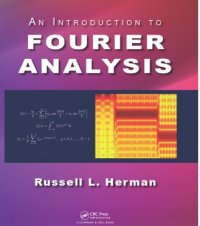Fourier and Complex Analysis Notes

Back

 An Introduction to Fourier  Analysis,2016 by Dr. R. L. Herman

This introductory textbook was written with mathematics, science, and engineering students with a background in calculus and basic linear algebra in mind. It can be used as a textbook for undergraduate courses in Fourier analysis or applied mathematics which cover Fourier series, orthogonal functions, Fourier and Laplace transforms, and an introduction to complex variables. These topics are tied together by the application of the spectral analysis of analog and discrete signals, and provides an introduction to the discrete Fourier transform. A number of examples and exercises are provided including implementations of Maple, MATLAB, and Python for computing series expansions and transforms.https://www.crcpress.com/An-Introduction-to-Fourier-Analysis/Herman/p/book/9781498773706

Top

E-Mail: Dr. Russell Herman Last Updated: December 16, 2016